Track your rental property performance for FREE

# Why successful rental property owners focus on cash-on-cash returnsby Brad Cartier, posted in Finances, Investment StrategyTry Stessa for FREE today

Cash on cash return is a simple – and extremely useful – financial calculation that real estate investors use regularly. Cash-on-cash return for real estate investors measures the amount of net cash flow a property is generating as a percentage of the total amount of cash invested. In fact, the cash on cash metric is so important that it gets its own chart on the Stessa dashboard.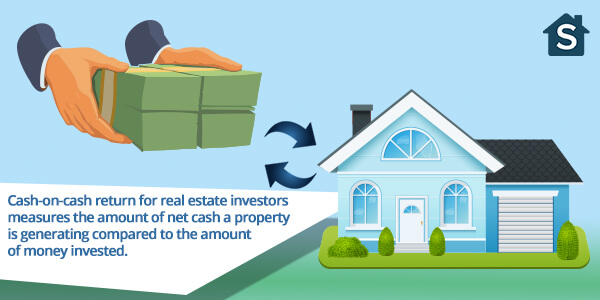Sometimes abbreviated as CoC or CCR, cash on cash is always expressed as a percentage and can be used to quickly compare the potential returns that different real estate investments offer.

## How do you calculate the cash-on-cash return for a rental property?

Figuring out the cash on cash percentage is relatively easy, but keep in mind that it’s usually expressed net of debt service and, at your option, can include or exclude principal debt payments. This essentially means there are two formulas for calculating the cash-on-cash return for a real estate investment:

The Low Road: Annual before-tax cash flow (but after all debt service) / Total cash invested = Cash-on-cash return

For instance, \$10,000 annual before-tax cash flow / \$100,000 total cash invested = 10% cash-on-cash return.

The High Road: Annual before-tax cash flow (but after interest payments only) / Total cash invested = Cash-on-cash return (with principal)

For instance, \$10,000 annual before-tax cash flow + 2,000 principal debt payments / \$100,000 total cash invested = 12% cash-on-cash return.

## Why is tax excluded from the cash-on-cash calculation?

The tax in the cash-on-cash calculation refers to the investor’s specific tax situation. The CoC from an investment property is the same regardless of who owns it, but the amount of income tax paid differs from investor to investor. Excluding tax from the calculation makes it easier to make an apples-to-apples comparison of different outcomes across real estate investments (and investors).

## Is cash on cash return the same thing as cash flow?

Cash-on-cash return is different from cash flow in two ways:

• First, as shown in the example above, cash-on-cash is expressed as a percentage while cash flow is expressed as an amount.
• Second, cash flow shows you how much money you’ll have available at the end of the day to deposit into your bank account after all of your expenses have been paid (except income tax). Cash-on-cash tells you what kind of return you’re receiving for the total amount invested (acquisition equity plus subsequent equity infusions).

Here’s another way to think about CoC compared to cash flow. Let’s say you deposit that same \$100,000 in a CD. According to BankRate.com the current rate is about 2.7% and you’d receive \$2,700 each year. So, \$2,700 is your annual cash flow and 2.7% is your (somewhat shabby) cash-on-cash return.

## 5 examples of how to use a cash-on-cash return

Now, let’s look at five examples of how cash-on-cash returns are calculated in different real estate investment scenarios:

### Example #1

Property purchased for \$250,000 all cash with \$25,000 annual cash flow: \$25,000 / \$250,000 = 10% cash-on-cash return.

### Example #2

Property purchased for \$250,000 all cash + \$50,000 in repairs and \$25,000 annual cash flow: \$25,000 / \$300,000 = 8.3% cash-on-cash return.

### Example #3

Property purchased for \$50,000 down with \$10,000 annual cash flow after debt service: \$10,000 / \$50,000 = 20% cash-on-cash return.

### Example #4

The property in the above example #3 needs a new HVAC system, total cost \$4,000. Cash-on-cash return for this year is \$6,000 / \$50,000 = 12%. Note that in this example you don’t add the \$4,000 capital expense to the denominator because you covered the cost out of cash flow, not new equity.

### Example #5

In the second year of owning the property discussed in example #3, let’s assume rents are increased by 10%, resulting in a 15% increase to net cash flow. Remember that if rents go up by more than expenses, you’ll get a multiplier effect on net cash flow. Cash-on-cash return for the second year then becomes \$11,500 / \$50,000 = 23% versus the 20% we saw during the first year of example #3.

## How is cash-on-cash different from other real estate investing metrics?

We’ve already discussed the difference between CoC and cash flow, how to calculate cash on cash, and what the cash-on-cash return calculation is used for. Now, let’s look at some of the other common real estate financial metrics used to evaluate real estate asset performance:

### NOI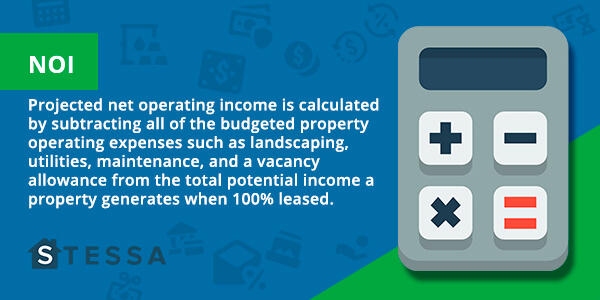Projected net operating income is calculated by subtracting all of the budgeted property operating expenses such as landscaping, utilities, maintenance, and a vacancy allowance from the total potential income a property generates when 100% leased. Debt service is not included as an expense when calculating NOI, whereas cash-on-cash includes the debt service expense.

### Cap Rate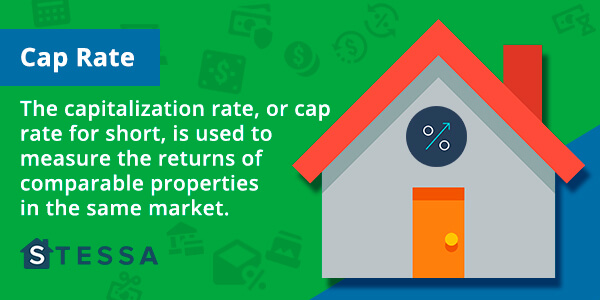The capitalization rate, or cap rate for short, is used to measure the returns of comparable properties in the same market. The cap rate calculation is: NOI / market price (or current valuation) = cap rate %. If a property’s market price is \$1,000,000 and the NOI (before debt service) is \$60,000, the cap rate would be 6%.

By manipulating the equation, the cap rate formula can also be used to solve for the market value of a property, based on the NOI and cap rates of similar properties. It can also be used to determine what the NOI should be based on the asking price of a property and the market cap rate, but this is a less common application since it’s really the valuation that should flex to meet the market based on the current NOI.

### IRR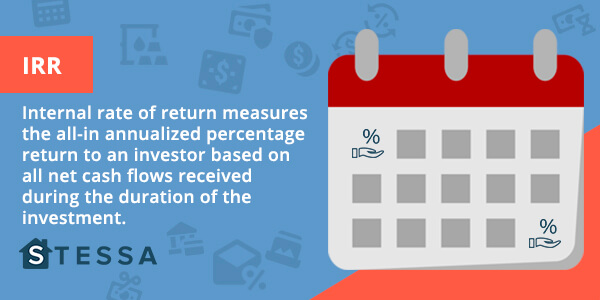Internal rate of return measures the all-in annualized percentage return to an investor based on all net cash flows received during the duration of the investment. Time is money, especially when investing in real estate. Generally speaking, the longer an investor’s money is tied up the lower the IRR will be and the worse the investment will perform.

The formula for calculating IRR is complex and requires the use of a financial calculator, Excel spreadsheet, or specialized calculator through a Google search.

### ROI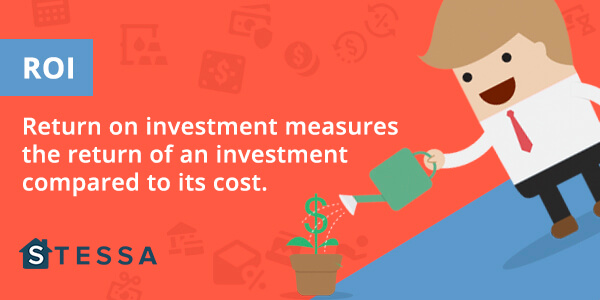Return on investment measures the return of an investment compared to its cost. The ROI formula is: (Current market value – Cost of investment) / Cost of investment. If we paid \$500,000 for a property last year, incurred no capital expenses, and today it is worth \$600,000, the ROI would be:

((\$600,000 – \$500,000) = \$100,000) / \$500,000 = 20%

If a property generates income, or it is held longer than one year, investors should factor this into calculating the return on investment to create a clearer picture of the ROI.

## FAQs about cash on cash returns

Q: What is a good cash-on-cash return?

A: It depends on the investor, the local market, and your expectations of future value appreciation. Some real estate investors are happy with a safe and predictable CoC return of 7% – 10%, while others will only consider a property with a cash-on-cash return of at least 15%.

Q: Is cash on cash the same as ROI?

A: No. ROI is used to measure the overall rate of return on a property including debt and cash, while cash on cash measures the return on the actual cash invested.

Q: Is cash on cash return the same thing as the cap rate?

A: If there’s no debt service (and no capital expenses) on the property then the cash on cash return would be the same as the cap rate. However, because real estate investors usually use the power of leverage, cap rates are almost always different from cash on cash returns.

## Key takeaways about cash-on-cash returns

As real estate investors we have a lot of metrics to choose from when analyzing properties and pitching money partners. CoC return is one of many key metrics that will help showcase a property’s potential return in an easily comparable number. Generally speaking, the higher the percentage of cash-on-cash return the better the real estate investment. Here are some other key takeaways to consider when using this important metric:

• Cash-on-cash return is a quick real estate financial calculation used to measure the percentage of cash received in a given month or year compared to total cash invested.
• Cash on cash is expressed as a percentage while actual cash flow is expressed as a dollar amount.
• Debt service is included in one version of the cash-on-cash return calculation, but it’s not included when calculating NOI or the cap rate.